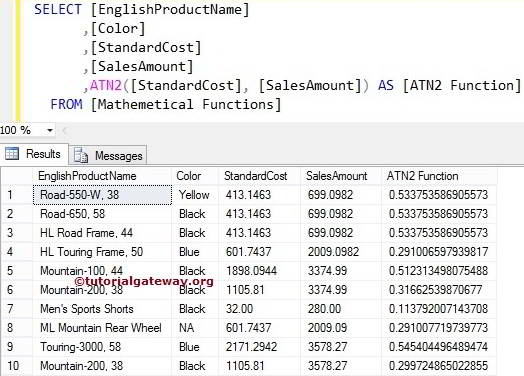# SQL ATN2 Function

The SQL ATN2 function is a Mathematical Function that returns the angle between the positive x-axis and the line from the origin to the point (y, x). The syntax of the SQL Server ATN2 Function is.

```SELECT ATN2 (Float_Expression, Float_Expression)
FROM [Source]```

For this ATN2 Function demo, we use the Math Table data

## SQL ATN2 Function Example 1

The ATN2 function finds the angle between the positive x-axis and the line from the origin to the point (y, x). Here, the x and y values are the two-argument we are going to provide for the ATN2 function. The following Mathematical Function query shows multiple ways to use the ATN2 function.

```DECLARE @i Float = 50.05, @j Float = 200.05

SELECT ATN2(@i, @j) AS [ATN2 Function]

-- Calculating ATAN directly
SELECT ATN2(100.27, 50.27) AS [ATN2 Function]

-- Calculating ATAN directly
SELECT ATN2(22.45 + 77.55, 100) AS [ATN2 Function]
```

We used the ATN2 function to find the angle between @i and @j and assigned a new name ‘ATN2 Function’ using ALIAS.

`SELECT ATN2(@i, @j) AS [ATN2 Function]`

## ATN2 Function Example 2

In this SQL example, we will calculate the angle between the values present in [Standard Cost] and [Sales Amount].

```SELECT [EnglishProductName]
,[Color]
,[StandardCost]
,[SalesAmount]
,ATN2([StandardCost], [SalesAmount]) AS [ATN2 Function]
FROM [Mathemetical Functions]```Categories SQL